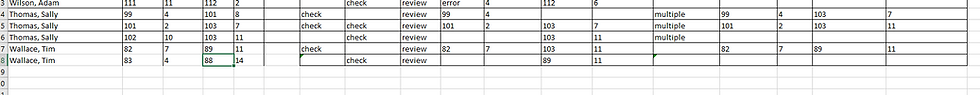top of pageSearch

# Find and Correct Overlapping Deposition Designations

You can use the spreadsheet attached below to identify and correct overlapping deposition designation page and line ranges.

Begin by entering the beginning and ending page and line ranges in columns B to E, listing the deponent's name in column A.. It is necessary to have the ranges sorted by deponent name, beginning page number, beginning line number, ending page number, and then ending line number.Next, pull down the formulas in columns F to I. The formula in G will find where an overlapping range begins, and the formula in H will find where it ends The formula in column will give a result of 'Review' for the ranges you need to correct, and blank for the ranges which can be left uncorrected in the final Excel file. The formula result in column F shows which ranges are simply duplicates.Filter in column G for 'check', and then pull down the formulas in J to R.

Then filter in column H for check, and pull down the formulas in columns J to R.The result will show the correct ranges on the same row as the beginning range in columns O to R.The formula in column N will show where more than two ranges overlap with one another.

Overlapping Depo Designations v2
.xlsx